An Ecosystem of δ-Potentials - II Corporeal Structures in Blip   22nd December 2021 HOME "Physics is becoming so unbelievably complex that it is taking longer and longer to train a physicist. It is taking so long, in fact, to train a physicist to the place where he understands the nature of physical problems that he is already too old to solve them." - Eugene Wigner "The essential point is that, although coordinates are a powerful, and sometimes essential, tool in many calculations, the fundamental laws of physics can be expressed without the aid of coordinates; and, indeed, their coordinate-free expressions are generally elegant and exceedingly powerful." - Kip Thorne "Can one not go a step beyond Palatini and base a theory on affine connexion alone, which is after all the first and only one needed to obtain a basis for mathematical analysis?" - Erwin Schrödinger, Space-Time Structure, Cambridge University Press, 1950. *** Our objective is to introduce various physical structures corresponding to different information contents, in the j-space fabric.  Sometime ago we discussed how a macroscopic observer ObsM from IS|q=3 (q = 3 information space), would measure a shallow-well from a higher IS|q=2, as a δ-potential in the current IS|q=3 (also known as Aku's universe Blip).      In this blog, we shall continue this discussion for the formation of  corporeal structures due to delta potentials of different information contents within IS|q=3.  We note that the definition of a delta potential in j-space is correlative, based on the measurements made by an observer, as discussed in the previous blog.     We first however need to understand the concepts behind the measurement of time and the measurement of space, as performed by ObsM.  We can not take it for granted that time⊗space exist empirically, or it has a unique structure as we perceive it.      The arguments presented next, are independent of the q-value of IS.   We shall consider the physical measurements in the .  We also remind ourselves of the basic assumption behind the discrete measurement space or j-space: "Every entity, simultaneously an observer and an object, is in a state of measurement and will continue to measure until the measurement is complete." Building a physical space from the information space         The question we will try to answer here is whether the physical space, can exist without any information content, keeping in mind that in j-space the information can be obtained only by measurements?  Furthermore does the physical space combined with time contains all the information, which is there to be measured?      In Blip, these measurements use the electron-photon interaction defined by the fine-structure constant α (~1/137).  As an example, we will be discussing the very first measurement towards building the conventional time⊗space in Blip2.  (Note: These measurements are performed by ObsM.)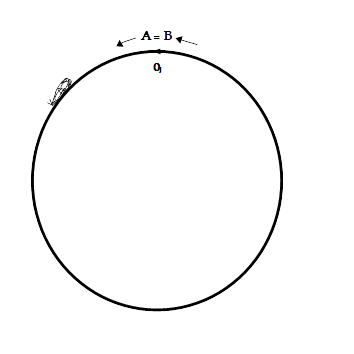The simplest measurement in j-space is equivalent to completing a circuit.  The observer obeys VT-Symmetry and arrives back at the point of origin.  Let us call the completed circuit, Path A-B.  Here A and B are identical points, i.e. the points A and B have same information contents.      Each e-γ interaction has a momentum associated with it, which is related to the physical space as a Fourier Transform, assuming that the  "Completeness" property is satisfied.  In j-space, the completeness property implies that with a given Hamiltonian, the path A-B can be measured with a complete precision.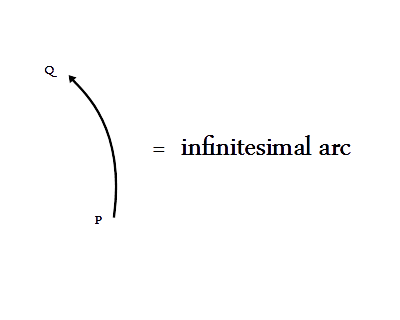We can also say that if an observer starts from point P on a path PQ, and arrives at Q, a measurement is completed.  We will be measuring paths A-B and/or PQ , using e-γ interactions.We note the truly brilliant induction experiment correlating relative motions of a physical body (magnet) and the magnetic coil connected to a source (charge/current).  Simply put, if we move magnet the current is induced in the magnetic coil and if we introduce a current in the magnetic coil the magnet is moved.  The end result for ObsM does not change in either case, except for the fact that a "relative" physical motion ( ≡  Path PQ), has taken place3.      Our objective is here to introduce the concept of the vector potential A, and the role it plays in the measurement of the infinitesimal path PQ.  The curl of A results in the magnetic field B, which by the definition of A, is a solenoidal field.  The presence of A allows an infinitesimal physical motion to take place when a Hamiltonian is activated.      In a sense, we are discussing the physical mechanism underlying the classical geometry using a discrete measurement space.  In geometry what is considered a single point, is actually the infinitesimal path PQ in j-space.  Therefore the time and the space are intricately connected to each other, and we are referring to this certitude symbolically as  "time⊗space".  The nature of infinitesimal path will be different for observers with different Hamiltonians.  Thus the description of time⊗space is strictly observer dependent.  Once we have the measurements of infinitesimal arcs, we can build various structures in the geometrical space as we discussed earlier. Some important features of the vector potential A, which are of interest to us in j-space, are:1. The vector field A is not measurable, however its curl is the magnetic field B which can be measured.  We can also find a scalar potential φ, which combined with A, gives us the electrical field E, which again is a measurable quantity.2.  The vector potential A, satisfies a wave equation under a certain condition known as Lorentz Gauge.  The wave equation is given as:Above equation, expressed in terms of the vector potential A and J, is an inhomogeneous wave equation.  The RHS of this equation represents the motion of charge (J = current density), which is a measurable quantity.       Therefore in principle, if we made the measurements in our lab in the presence of a charge distribution, by studying the nature of waves (incident and scattered), we can make predictions about the charge distribution's properties.  This is probably our earliest introduction to the topology underlying the physical laws of nature.      We note that photons represent the solution to the above equation in homogeneous form, i.e.Photons mean the polarization states, and the polarization states imply the formulation in terms of the creation and the annihilation operators. (This is an oversimplified version of the actual situation, since in this description the number of photons are assumed not to be conserved.  Please note that the total number of particles are conserved.  The condition of the particle conservation is enforced by fermions.)3.  We will notice that we are moving in the direction of the theory of Quantum ElectroDynamics (QED),  where we are interested in describing the interaction at a single point in time⊗space, as precisely as possible.      In QED, we have a moving charged particle described by the Schrödinger equation (HSch), we have electromagnetic fields described by the motion of photons (HEM), and we have the interaction between these two pictures (HInteraction).  The complete Hamiltonian, which represents the resources available to an observer performing the measurements in j-space, is written as:In HInteraction, the electric and magnetic field vectors, E and B,  are written in terms of the scalar potential  φ and the vector potential A, where A is assumed to satisfy the homogeneous wave equation.      We also note that HInteraction is invariant with respect to translations and rotations in time⊗space, as the nature of the e-γ interaction is universal for a given value of α.  In short if we can write a Hamiltonian, it means that the measurements based on this Hamiltonian provide a valid description of time⊗space. 4.  Assuming that we have been able to come up with a description of single one location in time⊗space, we have to ensure this description is consistent across the whole time⊗space in Blip.  We achieve this by the condition of "Locality".      The concept of Locality in essence, allows the Hamiltonian to be additive.  So the measurements at different locations on a given geometry in time⊗space add up and we can then write HTotal for Blip itself.  In ideal j-space, no two geometries in time⊗space will result in same HTotal, unless a symmetry is assumed.     The locality allows same measurement procedure to be true for all points in time⊗space, if the condition of locality does not hold it means that Hamiltonian is not additive, and the presented definition of the time⊗space is not valid.  It does not mean that the information-space does not exist, it just means that ObsM can not measure the information as time⊗space in Blip.  And without a valid description of  time⊗space, Blip can not exist. 5.  The Hamiltonian HTotal, is sum of all possible interactions present in a physical situation across different information spaces. Of which,  the measurements made using e-γ interactions form a very small subset.      For the description based on e-γ interactions, we consider the contributions from very low energies or very large wavelengths.  When we move to higher energies or shorter wavelengths, we move towards atomic and nuclear information domains.  These structures represent higher informations spaces, and hence greater resources are required to make predictions about the structures present in these domains.4     6.  Finally, we need to understand that the QED based description for time⊗space, is for an infinitesimal slice of time for which the ever increasing entropy of a discrete measurement space, is ignored.      However in reality that is not the case.  As the time progresses the information obtained by each e-γ interaction based measurement, is decreasing, whether an observer can measure this deterioration in α or not.  Subsequently at some point further down the time-axis, no more information will be obtained from the measurements, even though the time-axis for the observer itself may be infinite.  Thus the universe Blip as measured by Aku, is bounded.  It is also very evident that the information obtained in the beginning of the epoch ( t = 0+) by the measurements, is massive (α ~1).      Thus the entropy based limitations inherent in a measurement system, will restrict the time⊗space description obtained by this system.  Only way to improve the situation is to bring in new information into the system by reducing the q-value of IS.  This is equivalent to saying that ObsM needs to figure out how to improve the value of the fine-structure constant in Blip, which is our challenge.         We are discussing the measurements made by ObsM using the e-γ interactions, in IS|q=3.  Thus only corporeal structures are introduced in j-space fabric this time.  The ethereal structures based on higher information spaces exist as coherent states in j-space, as discussed previously.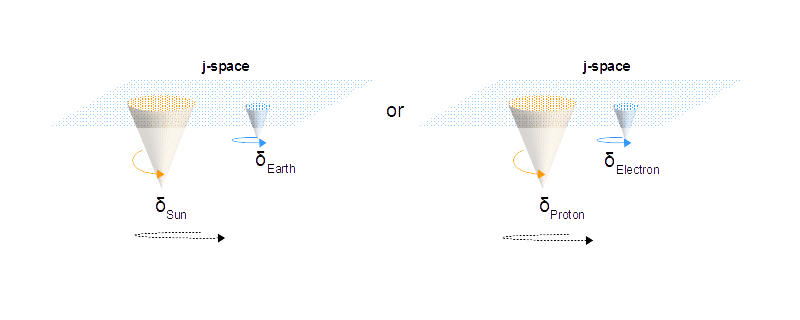We now have some intuitive understanding of the underlying time⊗space mechanism built into the j-space fabric, which is in the rest frame-S of ObsM.  Next we can start introducing information δ-functions, into this fabric.  Few facts:1.  The measurements to establish a geometrical space, are being made in the S-frame of reference, in which the macroscopic observer ObsM, is at rest.  The field(s) are established by the measurements made by force carriers, such as photons, mesons, gravitons, Higgs etc.  We assign a tangential entity, to the each point on the manifold formed by each of the force carriers.  The normal components in the field are necessitated by the presence of the δ-functions.  In the absence of δ-functions in the neighbourhood, the normal components are assumed to be infinitesimally small.  (Note:  A field in j-space cannot exist without the presence of at least one δ-function.) 2.  The δ-functions, are either singular or composite information structures which can not be completely measured by an observer with given resources.  Thus the concept of a δ-function in j-space is a correlative concept, based on an observer's Hamiltonian.  For example the δ-function deemed by ObsM, is not necessarily a δ-function for Obsc who has a much powerful Hamiltonian.3. The observers and objects, both are examples of δ-functions.  The infinite information source is the ultimate δ-function in j-space, which can not be precisely measured by Obsi, Obsc, or ObsM. 4.  Finite barriers represent the low-energy measurements in the region-I of Kruskal-Szekeres coordinates.  Similarly δ-potentials represent the high-energy measurements.  We are mainly interested in the structures formed by the measurements of δ-potentials made by the observer ObsM in the reference frame S.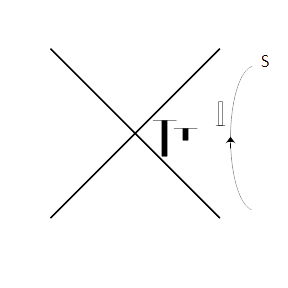Our discussion on the vector potential A will not be complete without a brief introduction to Kaluza-Klein theory.  In this theory we use a 4-vector vector potential Aμ = (φ/c, A) combined with a scalar field Φ,  to unify the field theories of gravitation and electromagnetism.  In this case a five dimensional metric tensor is written as,where gμν is the metric tensor from Einstein Field Equations.  This concept is also used in the string-theory as Compactification.   We will discuss this very elegant idea a little later in the context of j-space.     At present, our objective right now is to identify "the measurement" all observers in Blip are trying to make in a discrete measurement space.  In j-space, this agreement between observers defines the "unification" in the measurement space.  This particular discussion is far from over. _______________________ 1.  The q = 3 information space corresponds to α ~ 1/137. 2. We are not assuming the existence of either time or space.  Only assumption right now is that a macroscopic observer is measuring an infinite information source with α ~ 1/137. 3. If we could find a similar interaction mechanism in a different spin space, nobody said that we could not do the same with celestial bodies.  But then, that spin space has to be fermionic in nature. (Crazy stuff, please do not pay attention. Too much Star Trek!) 4. Furthermore, the Hamiltonian in the relativistic limits is not an invariant.  Which is not surprising as different information spaces overlap with each other under the relativistic limits. This creates a problem, which is resolved by noticing that the action is an invariant over a given path.  Therefore we use the Lagrangian formulation, which is easier to work with. We estimate the Hamiltonian next by integrating over all the possible trajectories for a given path, also known as the path integration formulation.  The Lagrangian formulation represents a dynamic measurement space, which is also the nature of j-space.  Therefore we seem to be in a reasonably good shape.   ***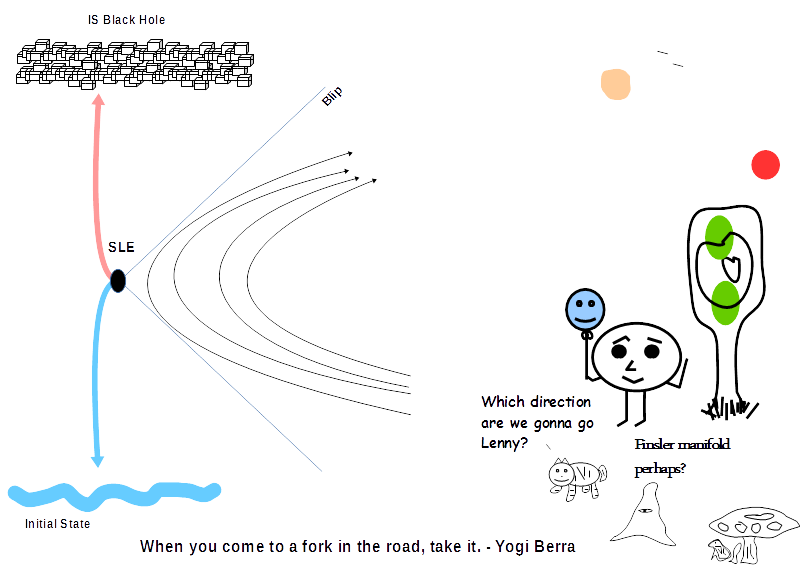Previous Blogs: EPR Paradox-I  Nutshell-2015Information on www.ijspace.org is licensed under a Creative Commons Attribution 4.0 International License. Attribution — You must give appropriate credit, provide a link to the license, and indicate if changes were made. You may do so in any reasonable manner, but not in any way that suggests the licensor endorses you or your use. No additional restrictions — You may not apply legal terms or technological measures that legally restrict others from doing anything the license permits. This is a human-readable summary of (and not a substitute for) the license.